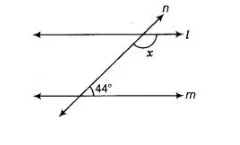# In the figure, find the value of x for which the lines l and m are parallel.`
Question:

In the figure, find the value of x for which the lines l and m are parallel.Solution:

In the given figure, l || m and we know that, if a transversal intersects two parallel lines, then sum of interior angles on the same side of a transversal

is supplementary. x + 44° = 180°

x = 180°-44°

=> x = 136° .